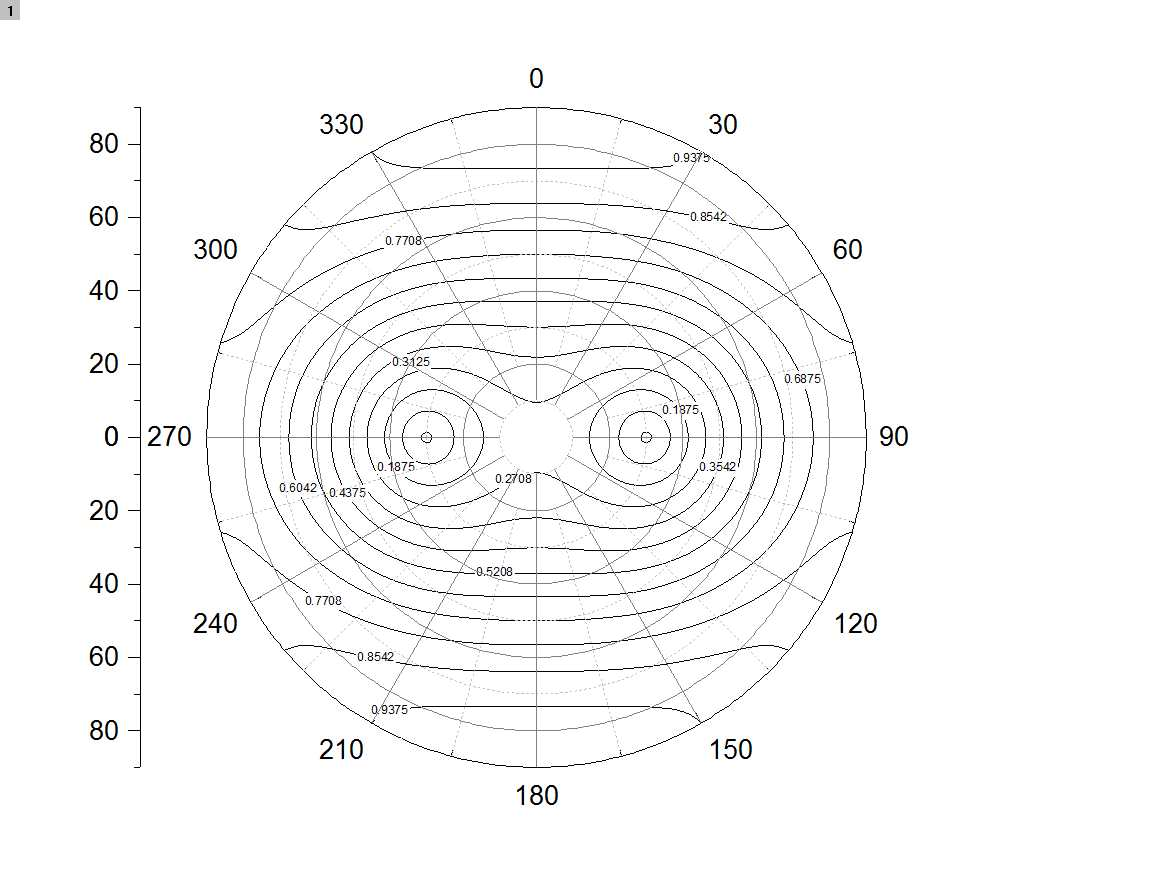# How to plot the contour polar map in matlab

72 views (last 30 days)
HEIN HTET ZAW on 31 Jan 2019
Edited: Adam Danz on 11 Oct 2020 at 17:42
Dear Mathlab users.
I am geoscience student, studying in University Technology PETRONAS, malaysia.
I need the help to plot the contour polar map,
I have X,Y,Z data
X= Azimuth ( 0 - 360) Theta
Y= Inclination ( 0 - 90) Radius
Z = values (0 - 1) contour line vales,
How can I process with this three data to get the contour polar plot.
I attached the csv input data and result of the plot using origin lab (example but the same as I want from Matlab),
Thank you and I appreciate for considering my problem.
Best Regards,
HeinAdam Danz on 1 Sep 2020
Unfortunately HEIN HTET ZAW has removed the content of this question which contained the demo data that made the answer useful to other people.
Copy of question
Dear Mathlab users.
I am geoscience student, studying in University Technology PETRONAS, malaysia.
I need the help to plot the contour polar map,
I have X,Y,Z data
X= Azimuth ( 0 - 360) Theta
Y= Inclination ( 0 - 90) Radius
Z = values (0 - 1) contour line vales,
How can I process with this three data to get the contour polar plot.
I attached the csv input data and result of the plot using origin lab (example but the same as I want from Matlab),
Thank you and I appreciate for considering my problem.
Best Regards,
HeinRena Berman on 9 Oct 2020 at 16:50
Adam Danz on 9 Oct 2020 at 22:45
Thanks Rena. But it looks like it's been deleted again. Since the data is used in my answer, maybe it could be attached to the answer.

Adam Danz on 31 Jan 2019
Edited: Adam Danz on 1 Feb 2019
Hmmmm, I'm not sure if it's possible to directly draw contour lines in polar coordinates with Matlab (I'd love to know otherwise). But here are some alternatives.
Use a scatter plot on polar coordinates.
polarscatter() allows for 3D inputs
% 'vals' are your values, 'az' azimuth, 'incl' inclination.
valScaled = round(vals*100);
colmap = colormap(jet(101));
figure
ph = polarscatter(az * pi/180, incl, [], colmap(valScaled+1,:), 'filled'); %convert to radians
colormap(colmap)
h = colorbar();
ylabel(h, 'value')Plot contour lines, but in Cartesian coordinates
% az are your azimuth data (column vector)
% incl are your inclination data (column vector)
% vals are your 3rd column of data.
azMat = reshape(az, length(unique(az)), []);
inclMat = reshape(incl, [], length(unique(incl)));
valsMat = reshape(vals, length(unique(az)), length(unique(incl)));
figure
contour(azMat, inclMat, valsMat)
xlabel('azimuth')
ylabel('inclination')
h = colorbar;
ylabel(h, 'values')Use a 3rd party function from the file exchange
There are several. I tried out the file exchange function polarcont() : https://www.mathworks.com/matlabcentral/fileexchange/14826-polar-contour-plot
This transforms the data from polar to cartesian coordinates and plots it in cartesian space.
valsMat = reshape(vals, length(unique(az)), length(unique(incl)));
figure
polarcont(unique(incl), unique(az * pi/180), valsMat', 20); %note conversion to radian & transpose!HEIN HTET ZAW on 1 Feb 2019
Sir Danz,
Is it possible to show the value by contour lines? I want to use contour polar map instead of using coloar map. As I shown the photo is the same as I want but I don't have idea to write script for it.
However, thank you and I appredicated for your helping.
Best Regards,
Hein
Adam Danz on 1 Feb 2019
I updated my answer to provide 2 more alternatives. If you find the solution you're looking for elsewhere, I'd be happy to see it.
HEIN HTET ZAW on 6 Feb 2019
Thank you so much for consideration sir Danz, your answer is quite useful for me.